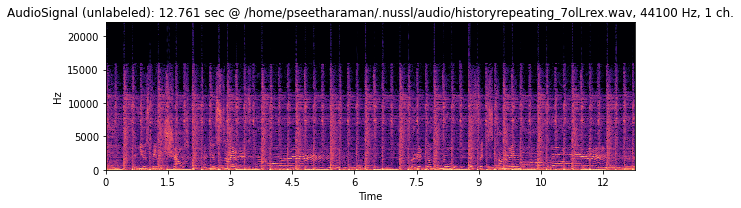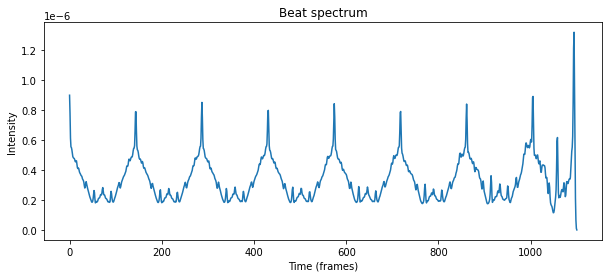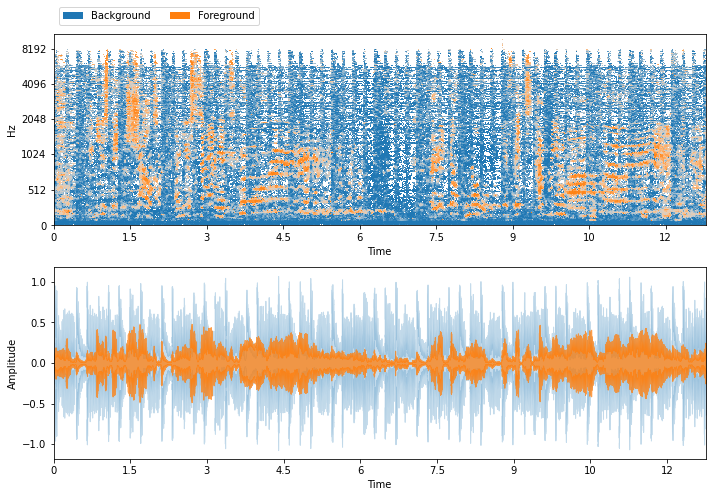# Running REPET¶

## Introduction¶

The REpeating Pattern Extraction Technique, or REPET, is source separation algorithm that separates a repeating “background” from a non-repeating “foreground”. REPET finds the repeating period in an audio signal, slices the signal into “frames” of the same length of the repeating period and “overlays” those frames. Once the frames are overlayed, REPET extracts the non-repeating part by filtering out values that are far from the median value at each frame.

In order to run REPET in nussl, we first must create an AudioSignal object. We’re going to load a file as before.

:

import nussl
import matplotlib.pyplot as plt
import time

start_time = time.time()

history = nussl.AudioSignal(audio_path)
history.embed_audio()

plt.figure(figsize=(10, 3))
nussl.utils.visualize_spectrogram(history)
plt.title(str(history))
plt.tight_layout()
plt.show()

Matching file found at /home/pseetharaman/.nussl/audio/historyrepeating_7olLrex.wav, skipping download.Neat. Now, we need to instantiate a Repet object. Like all source separation algorithms in nussl, Repet needs an AudioSignal object as its first parameter when we initialize it.

:

repet = nussl.separation.primitive.Repet(history)


Like all other algorithms in nussl, Repet has made its own copy of our history object that it will manipulate, so we can reuse history again if we want to. Any modifications made on the signal passed to Repet will be done on a copy of history.

## Repeating Period¶

If we know exactly what the repeating period is, we can give that Repet or if we kind of know where it is we can give it some estimates. Say I think the repeating period is about 3.5 seconds.

:

repet_exact_period = nussl.separation.primitive.Repet(
history, period=3.5)  # exact period
repet_period_guess = nussl.separation.primitive.Repet(
history, min_period=3.4, max_period=3.6)  # guess the period


But! If we have no clue, then Repet will try to find the repeating period for us, automatically. So we’re back to this:

:

repet = nussl.separation.primitive.Repet(history)


## Running Repet¶

Now, we can run the algorithm, and all nussl algorithms, in a few ways. Every nussl algorithm has two important functions:

1. run()

2. make_audio_signals()

The first one runs all of the necessary things to make the algorithm work. If it’s a masking-based algorithm, then run() returns a list of SoftMask or BinaryMask objects. The second actually creates the audio signals that correspond to the separated sources.

But if we do make_audio_signals before doing run, this happens:

:

estimates = repet.make_audio_signals()

---------------------------------------------------------------------------
SeparationException                       Traceback (most recent call last)
<ipython-input-5-d5a357b6d678> in <module>
----> 1 estimates = repet.make_audio_signals()

219             raise SeparationException(
--> 220                 "self.result_masks is empty! Did you call self.run()?")
221
222         estimates = []

SeparationException: self.result_masks is empty! Did you call self.run()?


So we should do run first:

:

masks = repet.run()

[<nussl.core.masks.soft_mask.SoftMask object at 0x7f5957d5d550>, <nussl.core.masks.soft_mask.SoftMask object at 0x7f59d8d69690>]


then we can do:

:

masks = repet.run()
estimates = repet.make_audio_signals()


Or we can chain both operations together by calling the object directly, like so:

:

estimates = repet() # does run then make_audio_signals


Now Repet has been run, so we can check out properties of Repet.

:

repet.repeating_period

:

287

:

plt.figure(figsize=(10, 4))
plt.plot(repet.beat_spectrum)
plt.xlabel('Time (frames)')
plt.ylabel('Intensity')
plt.title('Beat spectrum')
plt.show()We can see a regularly repeating period happening. REPET uses this periodicity to separate the background (repeating) from the foreground (non-repeating).

## Output of REPET¶

Okay, okay, okay. Now that we’ve run Repet let’s listen to the sources!

:

_estimates = {
'Background': estimates,
'Foreground': estimates
} # organize estimates into a dict

plt.figure(figsize=(10, 7))
plt.subplot(211)
_estimates, db_cutoff=-60, y_axis='mel')
plt.subplot(212)
nussl.utils.visualize_sources_as_waveform(
_estimates, show_legend=False)
plt.tight_layout()
plt.show()

nussl.play_utils.multitrack(_estimates):

end_time = time.time()
time_taken = end_time - start_time
print(f'Time taken: {time_taken:.4f} seconds')

Time taken: 6.6235 seconds